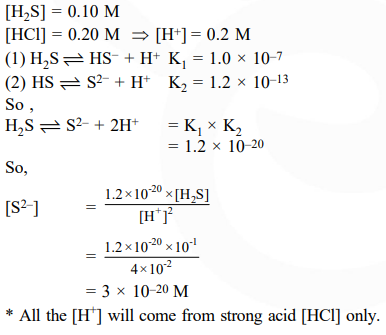# An aqueous solution contains

Question:

An aqueous solution contains $0.10 \mathrm{M} \mathrm{H}_{2} \mathrm{~S}$ and $0.20 \mathrm{M} \mathrm{HCl}$. If the equilibrium constants for the formation of $\mathrm{HS}^{-}$from $\mathrm{H}_{2} \mathrm{~S}$ is $1.0 \times 10^{-7}$ and that of $\mathrm{S}^{2-}$ from $\mathrm{HS}^{-}$ions is $1.2 \times 10^{-13}$ then the concentration of $\mathrm{S}^{2-}$ ions in aqueous solution is :

1. $3 \times 10^{-20}$

2. $6 \times 10^{-21}$

3. $5 \times 10^{-19}$

4. $5 \times 10^{-8}$

Correct Option: 1

Solution: